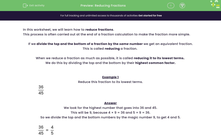# Use Common Factors to Simplify Fractions

In this worksheet, students will use common factors to simplify fractions.Key stage:  KS 2

Curriculum topic:   Number: Fractions, Decimals and Percentages

Curriculum subtopic:   Use Common Factors

Popular topics:   Factors worksheets

Difficulty level:#### Worksheet Overview

In this worksheet, we will learn how to simplify fractions

This process is often carried out at the end of a fraction calculation to make the fraction more simple.

If we divide the top (the numerator) and the bottom (the denominator) of a fraction by the same number we get an equivalent fraction.

This is called simplifying a fraction.

When we simplify a fraction as much as possible, it is called reducing it to its lowest terms.

We do this by dividing the numerator and the denominator by their highest common factor.

Example 1

Simplify this fraction to its lowest terms

 36 45

We look for the highest number that goes into 36 and 45.

This will be 9, because 4 × 9 = 36 and 5 × 9 = 36.

So we divide the numerator and the denominator by the magic number 9, to get 4 and 5.

 36 = 4 45 5

We cannot simplify this any further, so the answer is:

 4 5

Example 2

Simplify this fraction to its lowest terms.

 64 96

Sometimes it is hard to find the highest common factor.

We look for any number that goes into 64 and 96.

This could be 8, because 8 × 8 = 64 and 12 × 8 = 96.

So we divide the numerator and the denominator by the magic number 8, to get 8 and 12.

 64 = 8 96 12

Now we can simplify again by looking for the highest number that goes into 8 and 12.

This is 4 because 2 × 4 = 8 and 3 × 4 = 12.

So we divide the numerator and the denominator by the magic number 4, to get 2 and 3.

 2 3

Let's have a go at the questions now.

### What is EdPlace?

We're your National Curriculum aligned online education content provider helping each child succeed in English, maths and science from year 1 to GCSE. With an EdPlace account you’ll be able to track and measure progress, helping each child achieve their best. We build confidence and attainment by personalising each child’s learning at a level that suits them.

Get started